# Book Value

Updated June 22, 2021

## What Is Book Value?

When referring to assets, the term book value means the original cost of an asset minus accumulated depreciation.

### What Is the Book Value of a Company?

The book value of a company is the company’s total assets minus its outstanding liabilities. It represents the total amount of equity it would be worth to its shareholders after liquidating all of its tangible assets and paying all of its liabilities. It's useful for determining the value of a company and is often expressed as book value per share.

## Are Book Value and Carrying Value the Same?

Yes, book value is also referred to as “carrying value.”

## Where to Find Book Value on the Balance Sheet

Yes. On balance sheets, assets are listed at their book value (which is the original cost of the asset minus accumulated depreciation).

When referring to a company, book value is the same as shareholders’ equity on the balance sheet, which is the difference between assets and liabilities (minus intangible assets).

## How to Calculate Book Value

To calculate the book value of an asset, you subtract its accumulated depreciation from its original cost.

To calculate the book value of a company, you subtract the value of its total liabilities and intangible assets from the value of its total assets.

## Book Value Formula

Use the following formula to calculate the book value of an asset: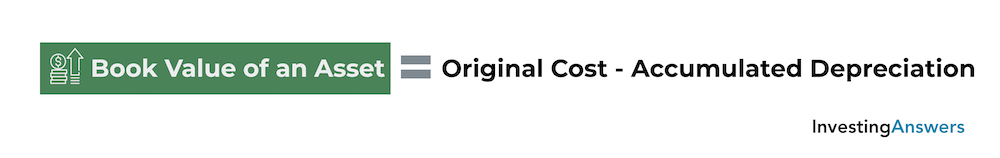Use the following formula to calculate the book value of a company: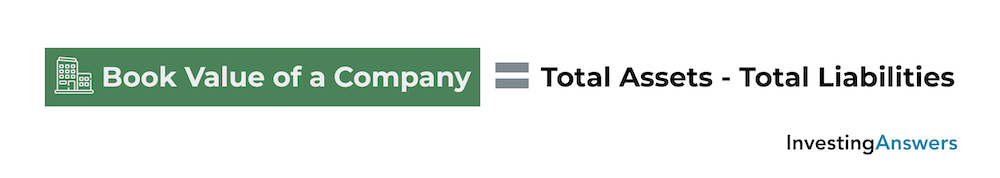### How to Calculate Total Assets

Use the following formula to calculate total assets: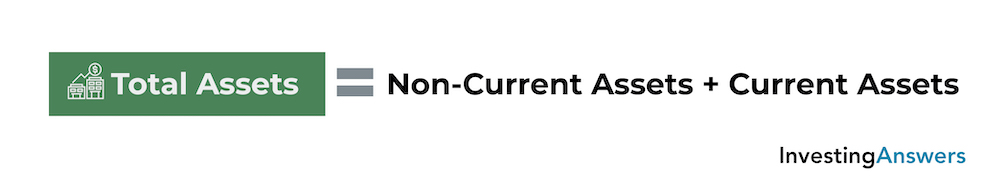### How to Calculate Total Liabilities

Use the following formula to calculate total liabilities: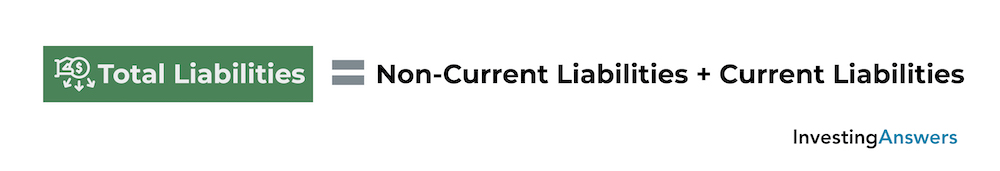## Why Book Value Is Important to Investors

Book value is important to investors because it provides an overview of a company’s total worth. This information has a number of applications:

## Limitations of Book Value

Despite its many benefits, book value does have some limitations:

### 1. Not Always Up to Date

Balance sheets are usually published quarterly or annually. Between reports, investors must rely on the latest figures, which often change from month to month.

### 2. Potentially complicated

In order to get an accurate book value, adjustments (e.g. depreciation) must be taken into account. There are different depreciation methods, accounting principles, and other considerations that can make calculations more complicated.

### 3. Intangibles Are Not Included

One of the biggest drawbacks to book value is that it doesn’t consider intangibles such as intellectual property or branding. Companies that rely heavily on human capital and intangible assets are much more difficult to value.

### 4. Ignores Growth

Assets and liabilities don’t always show the full picture. Companies that invest heavily in development or willingly operate at a loss can have a low or negative book value. If that figure is used to calculate the price-to-book-ratio, the ratio may (wrongfully) indicate that the company is undervalued or in distress.

### 5. Does Not Consider Quality

Book value does not consider the quality of a company’s assets, nor their current market price. Assets (like real estate) may gain value over time, while machinery and equipment can become outdated or less reliable. In both situations, the book value may not accurately reflect the value of the assets.

## What Does Book Value Per Share Mean?

Book value per share is a measure of the amount of equity that’s available to common shareholders on a per-share basis. In other words, it is the ratio of available common equity to the number of outstanding common shares. You can use the following formula to calculate book value per share: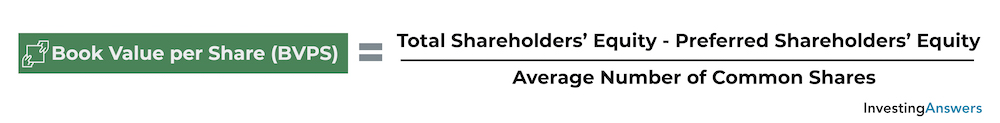Note: It is important to use the average number of common shares, as opposed to the total number of common shares at the end of a period. This is because major events like stock issuances or stock buybacks can affect the outcome of the calculation, erroneously making a stock seem over- or undervalued.

## What Is Book Value Per Share Used For?

Book value per share is another metric that investors use to analyze stocks. To determine whether stocks are undervalued or overvalued, investors can compare a company’s book value per share with the market value of its stock:

• If BVPS is higher than market value, the stock may be trading for less than the company is worth and is therefore undervalued.

• If BVPS is lower than market value, the stock may be trading for more than the company is worth and is therefore overvalued.

## What Book Value Per Share Is Considered Good?

Book value per share can vary significantly because every company is different. Factors such as assets, liabilities, and number of common shares can be influenced by company size, industry, and structure. There is no “good” or “bad” book value per share.

Book value per share is better used as a comparison tool. It can be used to compare against the current market value of a stock,  or against companies of similar size or within the same industry.

### Why Might Book Value Per Share Decrease?

Decreases in book value per share can occur when the numerator (common equity) decreases, or when the denominator (average number of common shares) increases. A decrease in common equity occurs when a company’s total assets decrease or total liabilities increase. On the other hand, an increase in average number of common shares occurs when a company issues stock.

## Book Value vs. Equity

While book value is the same as shareholders’ equity on a balance sheet, it is not the same as equity:

Book value is the company’s total assets minus its liabilities and intangible assets. It can be greater than, less than, or equal to zero.

Equity is the total value of all shares issued by a company and the value of all earnings that the company has retained. It can also be greater than, less than, or equal to zero.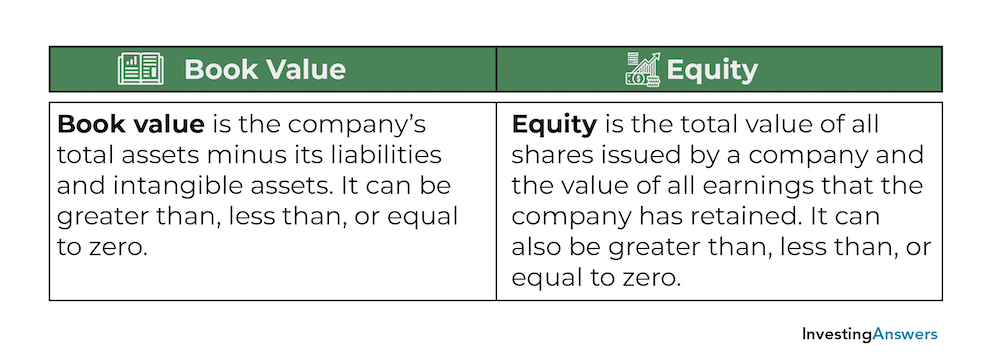## Book Value vs. Fair Value

Book value and fair value are both used to place a value on an asset, but the difference lies in the way that price is determined:

Book value is the carrying value of an asset, which is its original cost minus depreciation, amortization, or impairment costs. It is an estimate of what the asset is worth on the company’s balance sheet – but it doesn’t always reflect the actual price that it could be sold for.

Fair value is a reasonable estimate of the potential market value of an asset, determined by the price agreed upon by buyer and seller.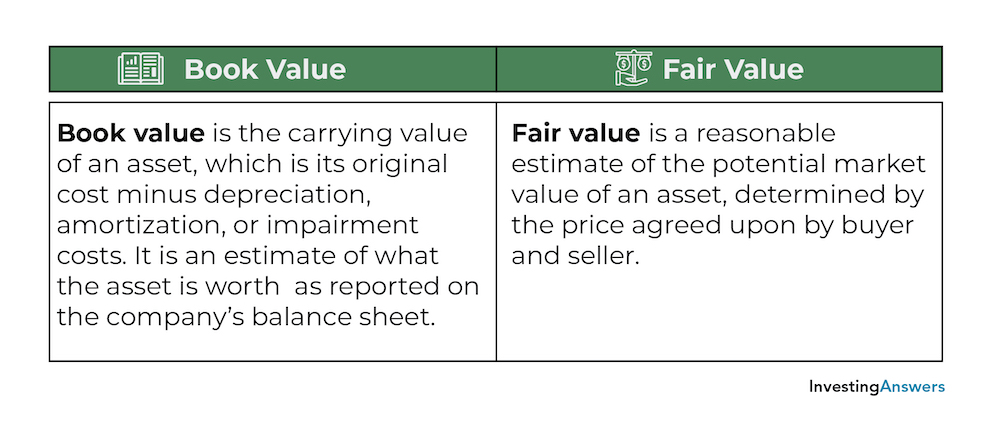## Book Value vs. Salvage Value

Book value and salvage value are two terms that refer to the value of an asset on a balance sheet with respect to depreciation. However, there is one major difference between book value and salvage value:

Book value (or carrying value) reports the value of an asset on a balance sheet, which is adjusted for depreciation.

Salvage value is the book value of an asset at the end of its useful life, once it has been fully depreciated.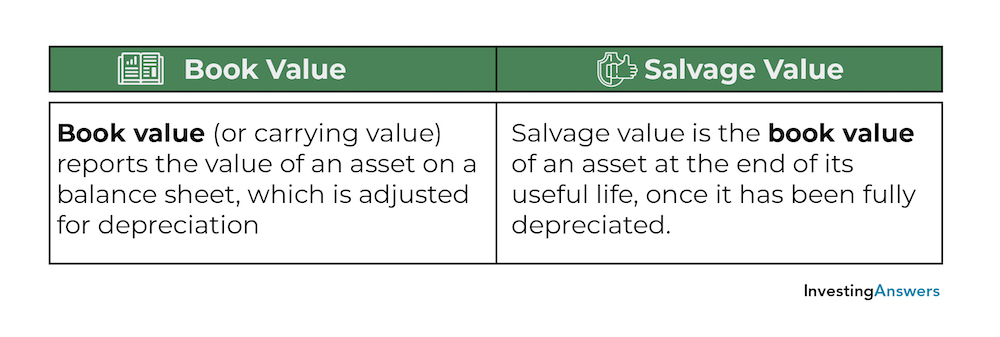All of our content is verified for accuracy by Rachel Siegel, CFA and our team of certified financial experts. We pride ourselves on quality, research, and transparency, and we value your feedback. Below you'll find answers to some of the most common reader questions about Book Value.

### Does Book Value Include Debt?

No. To obtain book value, liabilities (which include debt) and intangible assets are subtracted from total assets.

### Can Book Value Be Zero or Negative?

Yes. If total liabilities are the same as total assets, book value can be zero. When book value is negative, total liabilities exceed total assets.

### Are Book Value and Market Value the Same?

No. When referring to an asset, book value is the value of an asset on a balance sheet, minus the cost of depreciation. In this context, market value is the value of that asset in a marketplace.

When referring to a company, book value is the total value of a company if all of its assets were liquidated and all of its liabilities were paid off. It is equal to the total assets minus total liabilities and intangible assets. In this situation, market value is the total value of a company based on its current stock price and the number of shares. It is equal to the market price of its stock multiplied by its number of shares.

Rachel Siegel, CFA - 141Rachel Siegel, CFACFA Charterholder

Chartered Financial Analyst

Rachel Siegel, CFA is one of the nation's leading experts at ensuring the accuracy of financial and economic text.  Her prestigious background includes over 10 years creating professional financial certification exams and another 20 years of college-level teaching.